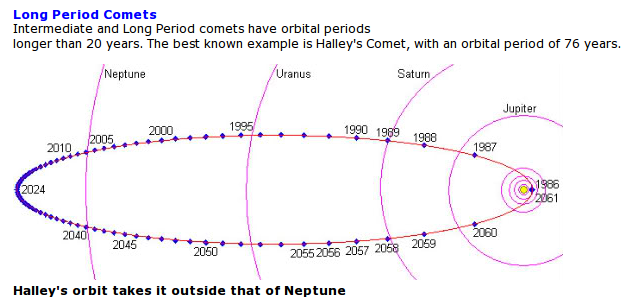## ISAAC NEWTON Math References

#### * BOOKS on Newton-math * WEBSITES on Newton-math * JOURNALS-and-MAGAZINES   with Newton-math articles

##### Sources that offer an expanded (or alternative) explanation of Newton's math results and methods.
###### (in English, rather than Latin or other languages)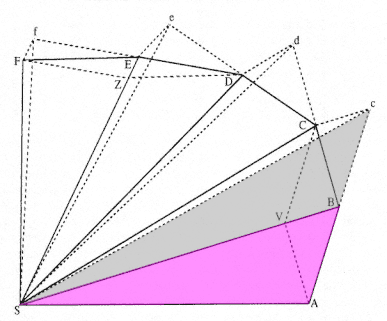Diagram for Theorem 1 of Book 1, Principia Inertial (constant velocity) flight in equal times : triangle areas SAB = SBc (because the triangles have equal bases and same height). Then imagine this inertial flight being pulled in by a continuously acting 'centripetal' force to a common center S. See figure to the right.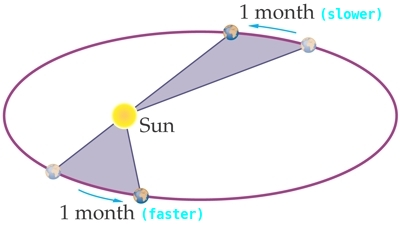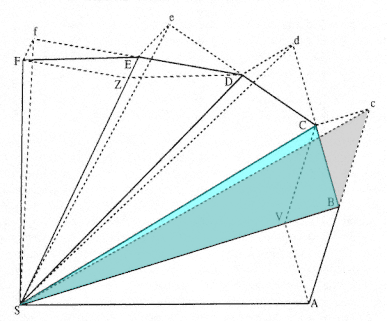Same diagram (of a discretized orbit) Centripital force effect, in the same time : triangle areas SBc = SBC (because the triangles have equal bases and same height). [BC is resultant of vectors BV (centripetal) and Bc (inertial).] Therefore triangle areas SAB = SBC (both = SBc). Similarly, SBC = SCD = SDE = SEF = ... equal area triangles in equal times. Thus, as time interval goes to zero, a radial from the force center S to an orbital curve sweeps out equal areas in equal times (think of two collections of extremely thin triangles) --- no matter where on the orbit --- and no matter what the form of the centripetal force, not just 'inverse-square' force. (Newton, the generalist, at work.) (Orbit could be elliptical, parabolic, hyperbolic, even spiral.) See figure to the left. You can imagine the two equal areas to be composed of an equal number of extremely thin 'equal-time-thin-triangles' and extremely thin 'equal-time-fatter-triangles' --- in the 'slower' and 'faster' areas, respectively.

! Preliminary !
More book/journal/magazine/website
and author information may be added ---
and more book/journal/magazine/website

Go to the Start of the List of Books
(Skip the following Introduction)

Go to the Start of the List of Websites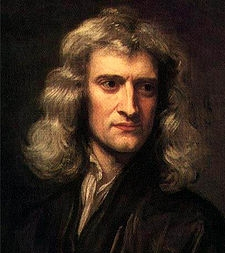One of the few portraits available of a youthful Newton.

START of LIST of Newton-Math BOOKS :
(in order by author last name)

START of LIST of Newton-Math JOURNALS/MAGAZINES :
(in order by publication name)

 Many of the following journals and magazines were mentioned in the references for the book 'Magnificent Principia' by Colin Pask. The publications that had about 5 or more articles on Newton's mathematics are indicated below by a double asterisk (**). Many of these journals do not allow open (free) access to their articles --- even articles that are more than 50 years old. So rather than providing a link to their web sites below, I provide a link to a web search on the journal/magazine name and keywords such as 'newton math'. If I ever find that some of the articles on Newton's mathematics are openly available from these journals, I may provide links to specific articles (of the journals) below.

START of LIST of Newton-Info WEBSITES :

 Newton Project at newtonproject.sussex.ac.uk (A website directed by Professor Rob Iliffe with the goal of compiling Newton's works into one electonic edition.) Stanford Encyclopedia of Philosopy at plato.stanford.edu (has some articles on Newton and the Principia --- for example, article by George E. Smith, 2007) National Library of Israel at web.nli.org.il (Many of the documents from a famous box of Newton's documents that sat essentially unopened at Cambridge for about 200 years were bought in a 1936 auction by this library. Mostly NON-mathematical papers.)

Bottom of this
Newton Math References page
--- books, journals, magazines, and web sites.

OR ...

##### < Go to Start of BOOKS List, above. > < Go to Start of JOURNALS/MAGAZINES List, above. > < Go to Start of WEBSITES List, above. > < Go to Top of Page, above. >

Page history

Page was created 2016 May 24.

Page was changed 2016 Jun 06.

Page was changed 2019 Mar 11.
(Added css and javascript to try to handle text-size for smartphones, esp. in portrait orientation.)

NOTE:
This web site may go dead before 2020.